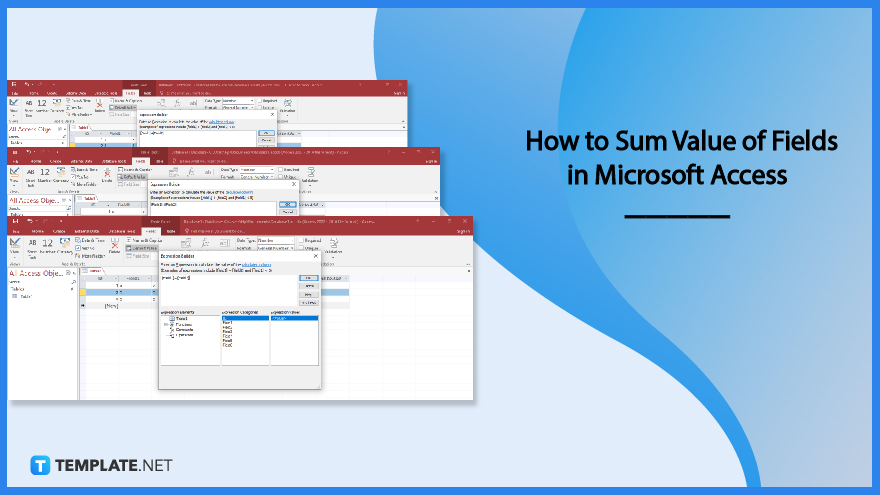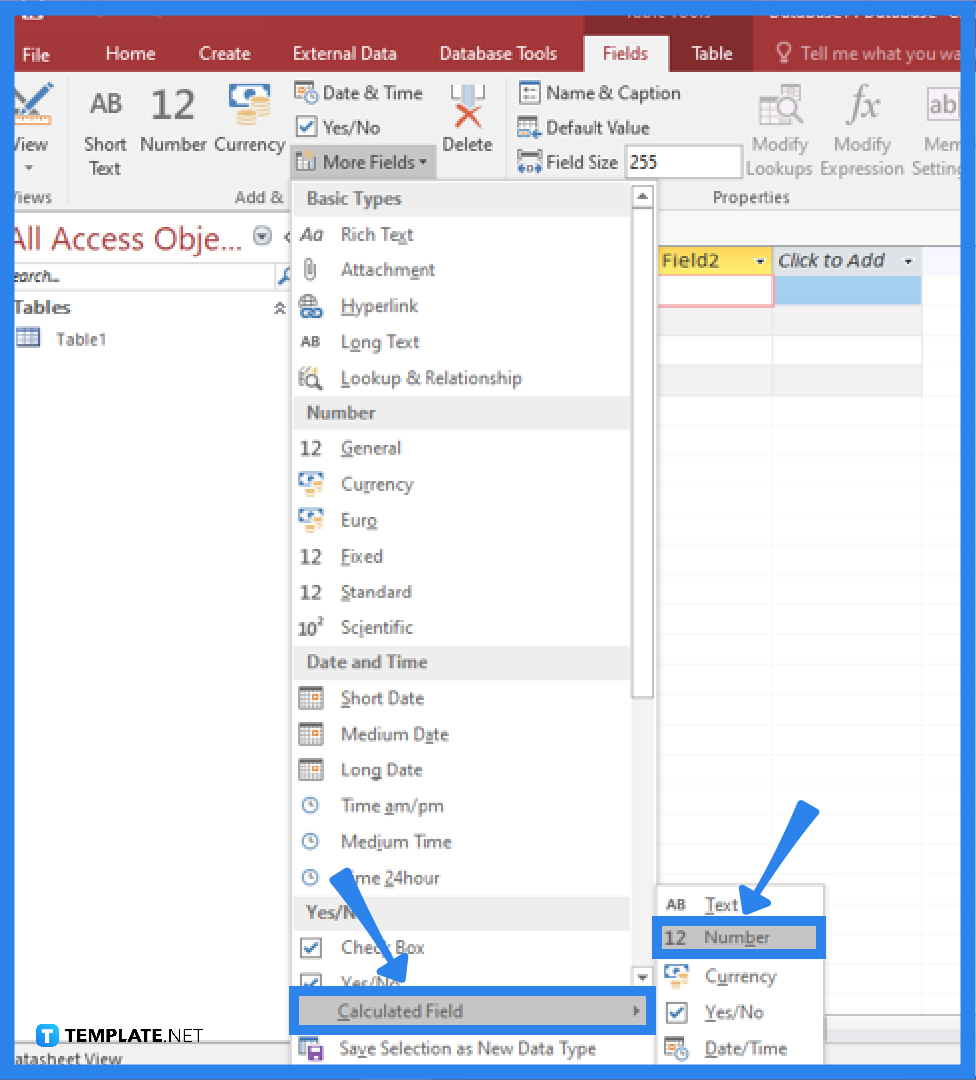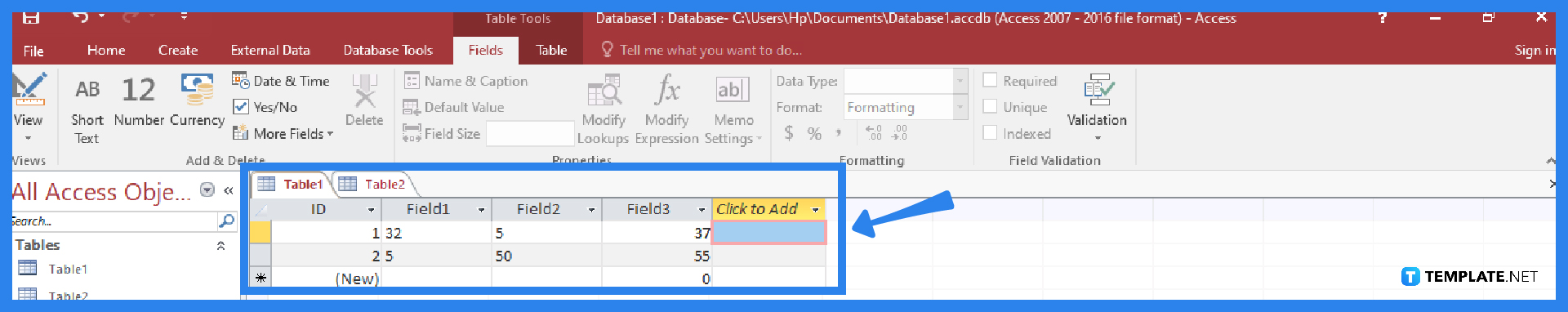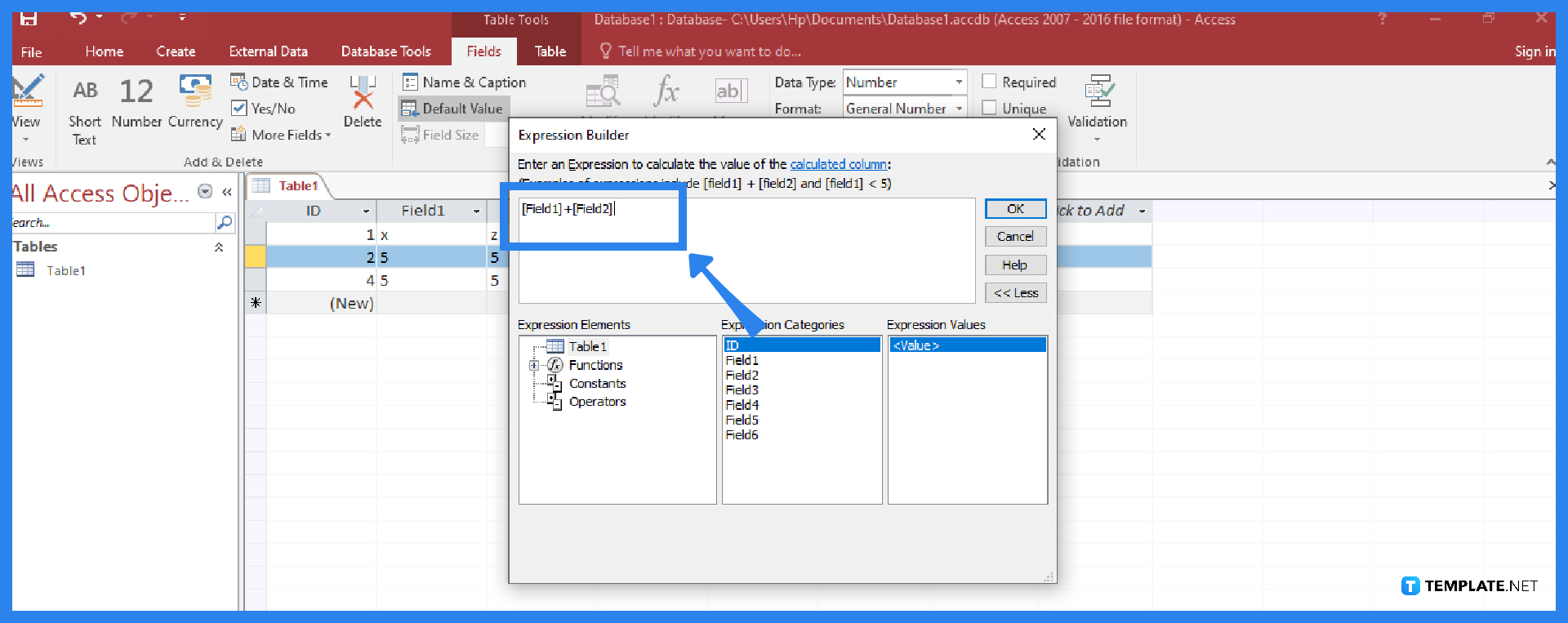# How to Sum Value of Fields in Microsoft Access

Calculated fields use information from a single record to make computations. In Microsoft Access, you can make calculations through the data in your tables using calculated fields and totals rows.## How to Sum Value of Fields in Microsoft Access

A calculated field is a new field that you can add that has calculations in each row involving other numerical fields in that row. You must enter a mathematical expression—a collection of your table‘s field names and mathematical symbols—to accomplish this.

• ### Step 1: Go to the Fields Tab in the Microsoft Access RibbonOpen Microsoft Access and enter the data to be calculated, or you can open an existing file to be calculated. Go to the Fields tab in the Access ribbon, locate the Add & Delete group, and click on the More Fields drop-down command.

• ### Step 2: Select Calculated FieldAfter clicking More Fields, a drop-down command will pop-up. Hover your mouse over the Calculated Field option and choose the data type to be calculated. The data type can be text, number, date/time, currency, or yes/no. As for the example, you should choose “Number” because you will perform the calculation as a number.

• ### Step 3: Construct the Mathematical ExpressionIt is now time to construct your mathematical expression. To select fields to include in your expression, double-click the field in the Expression Categories box. Don’t forget to add mathematical symbols like the plus sign. You must place the addition sign (+) between the fields because you want to find the sum of the values in the selected fields.

• ### Step 4: Finish the ProcessAfter you have finished writing your mathematical expression, click the OK button, and the calculated field will be added to your table. You can now sort or filter it as desired.

## FAQs

### What is a calculated field in Microsoft Access?

A calculated field makes it simple to calculate values from various table fields as well as from an Access built-in function, and the result of the calculation is then displayed in another field.

### How does the calculated field differ from the totals row in Microsoft Access?

Even though both the calculated field and the totals row let you perform calculations with the data in your tables in Microsoft Access, the totals row does a computation on an entire field of data, whereas calculated fields execute calculations using data from a single record.

### How is the value of a calculated field calculated in Microsoft Access?

Microsoft Access uses one or more additional fields in your table to determine the value of a calculated field, and these values in the calculated field are generated by Access each time the query is executed, never being saved anywhere.

### What do calculated expressions mean in Microsoft Access?

The value of a control can be retrieved, criteria can be added to a query, calculated controls and fields can be created, and group levels for reports can be defined are all operations that can be accomplished using expressions, and these calculated expressions include operators, constants, functions, and identifiers.